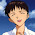## Combination Sum III

Find all possible combinations of k numbers that add up to a number n, given that only numbers from 1 to 9 can be used and each combination should be a unique set of numbers.
Ensure that numbers within the set are sorted in ascending order.

Example 1:
Input: k = 3, n = 7
Output:
[[1,2,4]]


Example 2:
Input: k = 3, n = 9
Output:
[[1,2,6], [1,3,5], [2,3,4]]

### Analysis:

This is quite similar to the previous problems Combination Sum I and Combination Sum II, please refer these two problems. Basically  DFS is enough to solve this problem.

### Code(C++):

class Solution {
public:
void search(vector<int> &candidates, int target, int i, int &k, vector<vector<int> >&res, vector<int> &ress){
if (target < 0){
return;
}else{
if (target == 0 && ress.size()==k){
res.push_back(ress);
}else{
for (int j=i;j<candidates.size();j++){
if (j==0 || candidates[j] != candidates[j-1]){
ress.push_back(candidates[j]);
search(candidates, target-candidates[j], j+1, k, res, ress);
ress.pop_back();
}
}
}
}
}
vector<vector<int>> combinationSum3(int k, int n) {
vector<vector<int> >res;
vector<int> ress;
vector<int> candidates(9);
for (int i=1;i<=9;i++){
candidates[i-1] = i;
}
search(candidates, n, 0, k, res, ress);
return res;
}
};


### Code(Python):

class Solution:
def search(self, candidates, target, i, k, res, ress):
if target < 0:
return
else:
if target == 0 and len(ress)==k:
res.append(ress[:]) #It is important to use "ress[:]" instead of "ress"
else:
for j in xrange(i, len(candidates)):
if j==0 or candidates[j] != candidates[j-1]:
ress.append(candidates[j])
self.search(candidates, target-candidates[j], j+1, k, res, ress)
ress.pop(-1) #if use "ress", this will pop the elemtnes in res also
# @param {integer} k
# @param {integer} n
# @return {integer[][]}
def combinationSum3(self, k, n):
res =[]
ress = []
self.search([1,2,3,4,5,6,7,8,9], n, 0, k, res, ress)
return res



1.2.3.